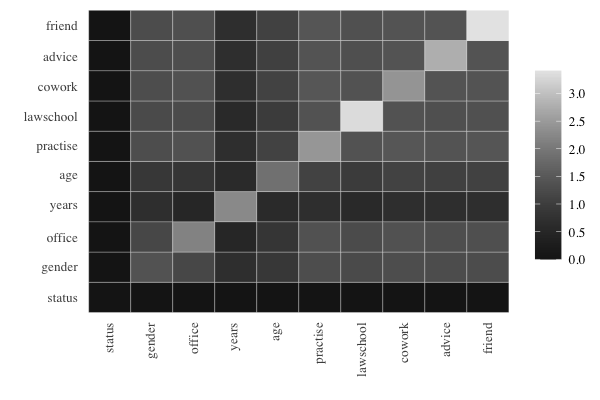# Prediction Power Based on Expected Conditional Entropies

In the section on univariate, bivariate and trivariate entropies, we saw that the bivariate entropy of two variables $$X$$ and $$Y$$ is bounded according to $H(X) \leq H(X,Y) \leq H(X)+H(Y) \ .$ The increment between the lower bound and the bivariate entropy is equal to the expected conditional entropy $EH(Y|X)=H(X,Y)-H(X)$ which is a measure of how far from functional dependence $$X\rightarrow Y$$ (which means that that $$X$$ uniquely determines $$Y$$) we are. This measure is equal to 0 if and only if $$p(x,y) = p(x,+)$$ meaning $$X$$ uniquely determines $$Y$$.

Similarly, trivariate entropies for triples of variables $$X,Y,Z$$ are bounded by $H(X,Y) \leq H(X,Y,Z) \leq H(X,Z) + H(Y,Z) - H(Z)$ and the increment between the trivariate entropy and its lower bound is equal to the expected conditional entropy given by $EH(Z|X,Y) = H(X,Y,Z)-H(X,Y)$ which is non-negative and equal to 0 if and only if there is functional dependence $$(X,Y)\rightarrow Z$$. Thus, $$EH(Z|X,Y)$$ measures the prediction uncertainty when $$(X,Y)$$ is used to predict $$Z$$.

$$EH=EH(Z|X,Y)$$ is a logarithmic measure of how many outcomes there are of $$Z$$ on average when the outcomes are given for $$X$$ and $$Y$$ . If $$EH$$ is rounded to its closest integer, we get an unambiguous prediction value for $$Z$$ based on predictors $$X$$ and $$Y$$ when $$EH < 0.5$$ and two prediction values for $$Z$$ when $$0.5\leq EH < 1.5$$ etc. Thus, prediction power is a decreasing function of $$EH$$.

## Example: prediction power based on expected conditional entropies

library(netropy)

We create a dataframe dyad.var consisting of dyad variables as described and created in variable domains and data editing. Similar analyses can be performed on observed and/or transformed dataframes with vertex or triad variables.

head(dyad.var)
##   status gender office years age practice lawschool cowork advice friend
## 1      3      3      0     8   8        1         0      0      3      2
## 2      3      3      3     5   8        3         0      0      0      0
## 3      3      3      3     5   8        2         0      0      1      0
## 4      3      3      0     8   8        1         6      0      1      2
## 5      3      3      0     8   8        0         6      0      1      1
## 6      3      3      1     7   8        1         6      0      1      1

The function prediction_power() computes prediction power when pairs of variables in a given dataframe are used to predict a third variable from the same dataframe. The variable to be predicted and the dataframe in which this variable also is part of is given as input arguments, and the output is an upper triangular matrix giving the expected conditional entropies of pairs of row and column variables of the matrix, i.e. $$EH(Z|X,Y)$$. The diagonal gives $$EH(Z|X)$$ , that is when only one variable as a predictor. Note that NA’s are in the row and column representing the variable being predicted.

Assume we are interested in predicting variable status (that is whether a lawyer in the data set is an associate or partner). This is done by running the following:

prediction_power('status', dyad.var)
##           status gender office years   age practice lawschool cowork advice
## status        NA     NA     NA    NA    NA       NA        NA     NA     NA
## gender        NA  1.375  1.180 0.670 0.855    1.304     1.225  1.306  1.263
## office        NA     NA  2.147 0.493 0.820    1.374     1.245  1.373  1.325
## years         NA     NA     NA 2.265 0.573    0.682     0.554  0.691  0.667
## age           NA     NA     NA    NA 1.877    1.089     0.958  1.087  1.052
## practice      NA     NA     NA    NA    NA    2.446     1.388  1.459  1.410
## lawschool     NA     NA     NA    NA    NA       NA     3.335  1.390  1.337
## cowork        NA     NA     NA    NA    NA       NA        NA  2.419  1.400
## advice        NA     NA     NA    NA    NA       NA        NA     NA  2.781
## friend        NA     NA     NA    NA    NA       NA        NA     NA     NA
##           friend
## status        NA
## gender     1.270
## office     1.334
## years      0.684
## age        1.058
## practice   1.427
## lawschool  1.350
## cowork     1.411
## friend     3.408
For better readability, the powers of different predictors can be conveniently compared by using prediction plots that display a color matrix with rows for $$X$$ and columns for $$Y$$ with darker colors in the cells when we have higher prediction power for $$Z$$. This is shown for the prediction of status:Obviously, the darkest color is obtained when the variable to be predicted is included among the predictors, and the cells exhibit prediction power for a single predictor on the diagonal and for two predictors symmetrically outside the diagonal. Some findings are as follows: good predictors for status are given by years in combination with any other variable, and age in combination with any other variable. The best sole predictor is gender.